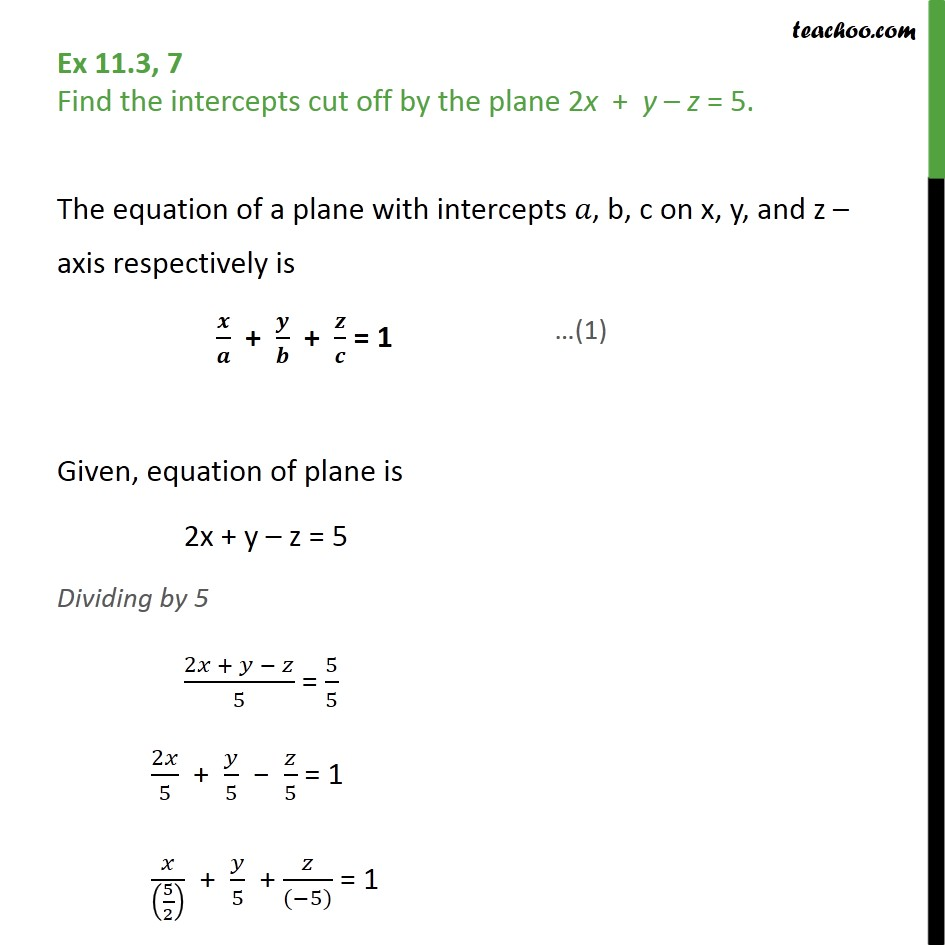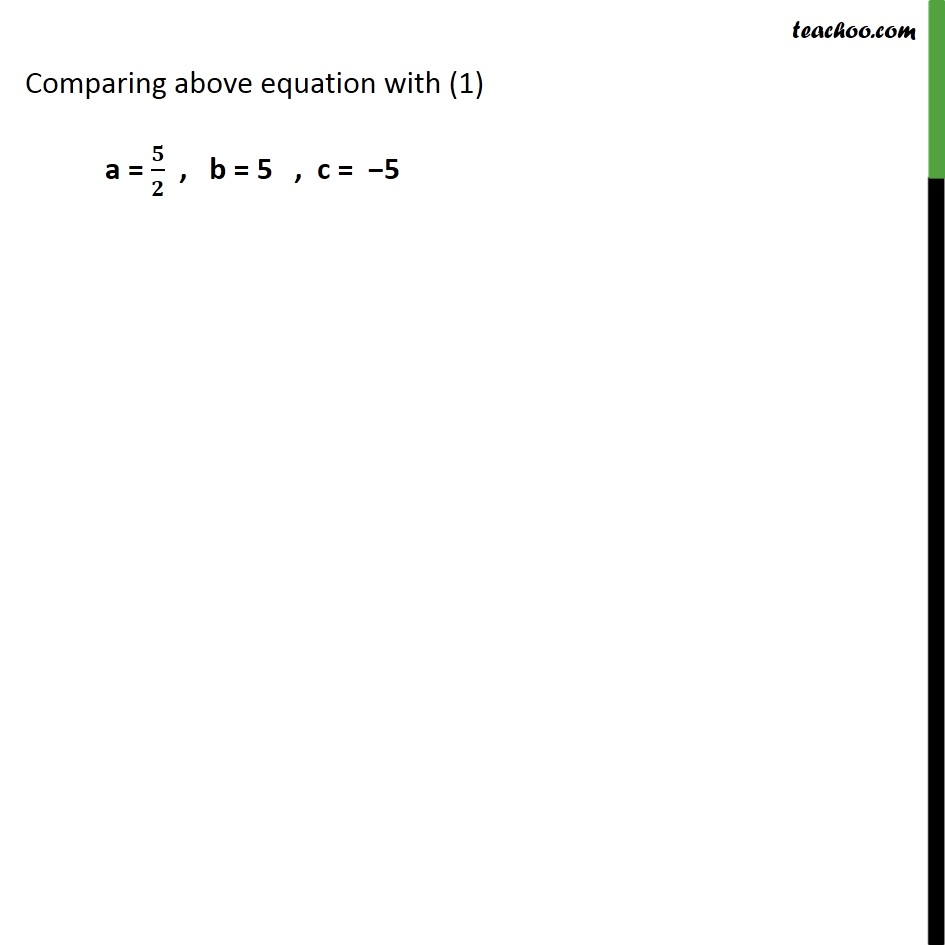Plane

Chapter 11 Class 12 Three Dimensional Geometry
Serial order wiseLearn in your speed, with individual attention - Teachoo Maths 1-on-1 Class

### Transcript

Question 7 Find the intercepts cut off by the plane 2x + y – z = 5. The equation of a plane with intercepts 𝑎, b, c on x, y, and z – axis respectively is 𝒙﷮𝒂﷯ + 𝒚﷮𝒃﷯ + 𝒛﷮𝒄﷯ = 1 Given, equation of plane is 2x + y – z = 5 Dividing by 5 2𝑥 + 𝑦 − 𝑧﷮5﷯ = 5﷮5﷯ 2𝑥﷮5﷯ + 𝑦﷮5﷯ − 𝑧﷮5﷯ = 1 𝑥﷮ 5﷮2﷯﷯﷯ + 𝑦﷮5﷯ + 𝑧﷮(−5)﷯ = 1 Comparing above equation with (1) a = 𝟓﷮𝟐﷯ , b = 5 , c = −5